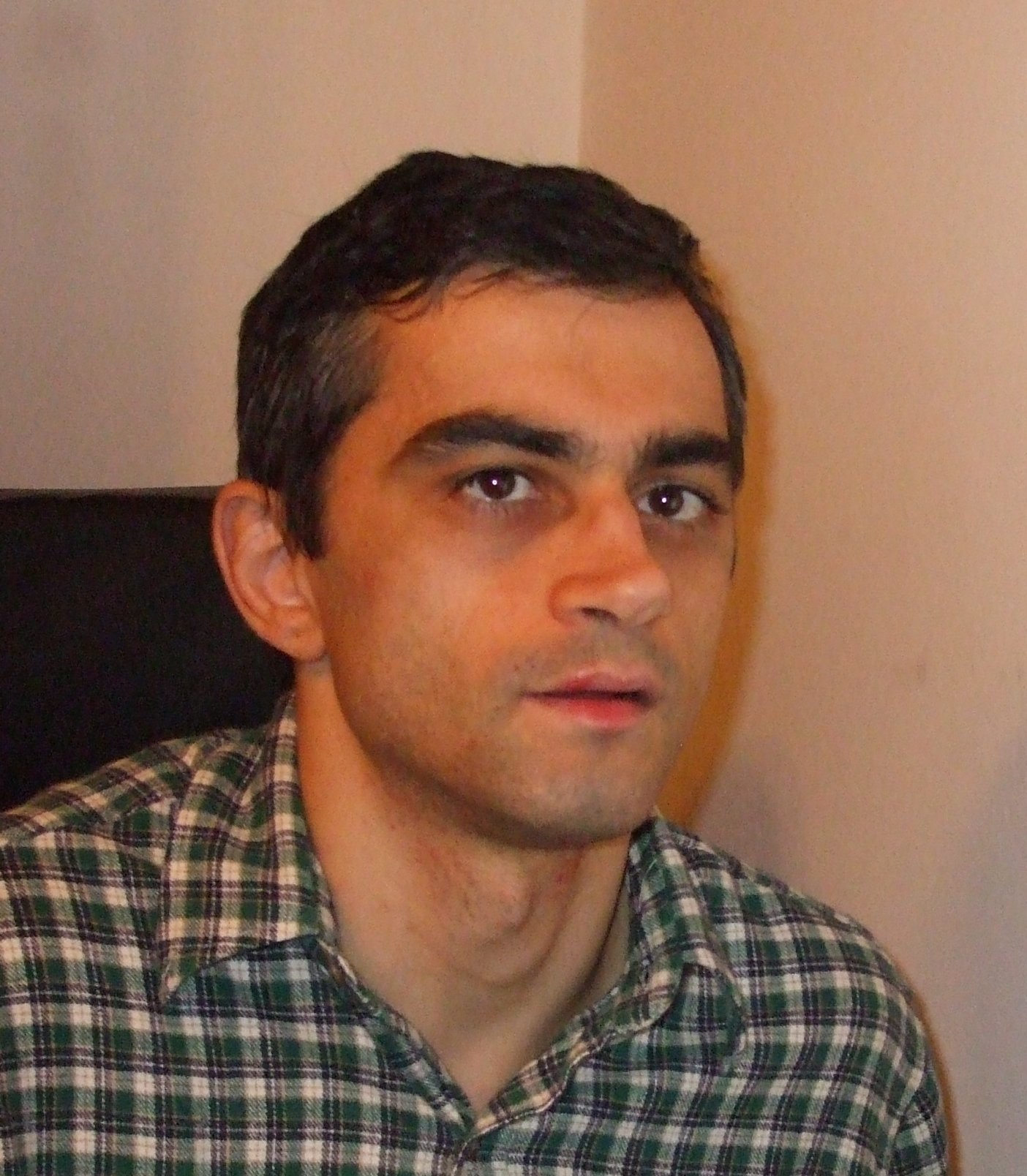## Beli Constantin-Nicolae

Institute of Mathematics of the Romanian Academy
P.O. Box 1-764, RO-70700 Bucharest, Romania

### Education

• Faculty of Mathematics, University of Bucharest, 1988-1993
• PhD, Ohio State University, 2001

### Employment

• 1993-present: Researcher, Institute of Mathematics "Simion Stoilow" of the Romanian Academy
• 1994-2001: Teaching Assistant, Ohio State University, Department of Mathematics

### Research interest

• Number Theory

### Scientific awards

• Prize "Simion Stoilow" of the Romanian Academy, 2003

### Scientifical Research

My most important results so far involve quadratic forms over dyadic local fields (finite extensions of $\Bbb Q_2$). To tackle problems in this area I introduced the notion of bases of norm generators (BONGs), which are a new way of describing the integral quadratic forms over dyadic local fields, complementary to the traditional Jordan decompositions. This new method allowed me to solve some old open problems, which previously had been solved only for nondyadic and 2-adic local fields (finite extensions of $\Bbb Q_p$ with $p>2$ and unramified finite extensions of $\Bbb Q_2$). These problems are:
1. Finding the integral spinorm norm group $\theta (O^+(L))$ of a quadratic lattice $L$.
2. Finding the relative integral spinorm norm group $\theta (X(M/N))$ associated to the quadratic lattices $N\subseteq M$.
3. Finding necessary and sufficient conditions such that a quadratic lattice represents another.
The solution to the first two problems above was given in my PhD thesis. The first one was published in my JNT paper in 2003. The solution to the third problem was announced in 2006 in my AMS-ERA paper. So far I have published a paper in the Transactions of the AMS (2010) containing a new version in terms of BONGs, of O'Meara's classification theorem 93:28, which is a pre-requisite for a larger paper, containing the solution to the representation problem which I have already sumbitted for publication.

### List of publications

1. "A new approach to classification of integral quadratic forms over dyadic local fields", Trans. Am. Math. Soc. 362, No. 3, 1599-1617 (2010).
2. "Two conjectures by Zhi-Hong Sun", Acta Arith. 137, No. 2, 99-131 (2009).
3. "Strong approximation of quadrics and representations of quadratic forms", J. Number Theory 128, No. 7, 2091-2096 (2008). (With Wai Kiu Chan.)
4. "Polynomials over ordered fields", Journal of Pure and Applied Algebra 209, No. 2, 507-517 (2007).
5. "Representations of integral quadratic forms over dyadic local fields", Electronic Research Announcements of the American Mathematical Society 12, 100-112, electronic only (2006).
6. "Integral spinor norm groups over dyadic local fields", Journal of Number Theory 102, No. 1, 125-182 (2003).

7. raspopitu1@yahoo.com
Beli.Constantin@imar.ro